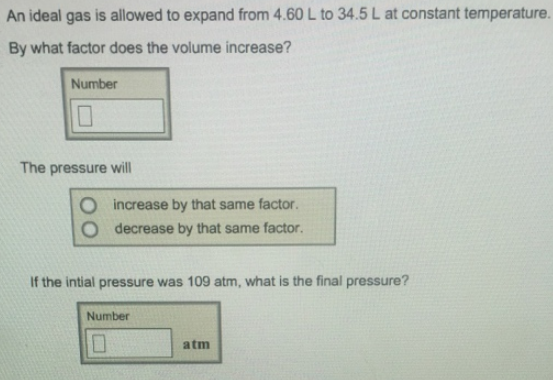# Problem: An ideal gas is allowed to expand from 4.60 L to 34.5 L at constant temperature. By what factor does the volume increase? The pressure will                        • increase by that same factor.                           • decrease by that same factor. If the initial pressure was 109 atm, what is the final pressure?

###### FREE Expert Solution
94% (124 ratings)###### Problem Details

An ideal gas is allowed to expand from 4.60 L to 34.5 L at constant temperature.

By what factor does the volume increase?

The pressure will

• increase by that same factor.

• decrease by that same factor.

If the initial pressure was 109 atm, what is the final pressure?